# Mutual information

## Mutual information API

The mutual information API is defined by

We provide a suite of estimators of various mutual information quantities. Many more variants exist in the literature. Pull requests are welcome!

## Mutual information definitions

CausalityTools.MIShannonType
MIShannon <: MutualInformation
MIShannon(; base = 2)

The Shannon mutual information $I^S(X; Y)$.

Discrete definition

There are many equivalent formulations of discrete Shannon mutual information. In this package, we currently use the double-sum and the three-entropies formulations.

Double sum formulation

Assume we observe samples $\bar{\bf{X}}_{1:N_y} = \{\bar{\bf{X}}_1, \ldots, \bar{\bf{X}}_n \}$ and $\bar{\bf{Y}}_{1:N_x} = \{\bar{\bf{Y}}_1, \ldots, \bar{\bf{Y}}_n \}$ from two discrete random variables $X$ and $Y$ with finite supports $\mathcal{X} = \{ x_1, x_2, \ldots, x_{M_x} \}$ and $\mathcal{Y} = y_1, y_2, \ldots, x_{M_y}$. The double-sum estimate is obtained by replacing the double sum

$$$\hat{I}_{DS}(X; Y) = \sum_{x_i \in \mathcal{X}, y_i \in \mathcal{Y}} p(x_i, y_j) \log \left( \dfrac{p(x_i, y_i)}{p(x_i)p(y_j)} \right)$$$

where $\hat{p}(x_i) = \frac{n(x_i)}{N_x}$, $\hat{p}(y_i) = \frac{n(y_j)}{N_y}$, and $\hat{p}(x_i, x_j) = \frac{n(x_i)}{N}$, and $N = N_x N_y$. This definition is used by mutualinfo when called with a ContingencyMatrix.

Three-entropies formulation

An equivalent formulation of discrete Shannon mutual information is

$$$I^S(X; Y) = H^S(X) + H_q^S(Y) - H^S(X, Y),$$$

where $H^S(\cdot)$ and $H^S(\cdot, \cdot)$ are the marginal and joint discrete Shannon entropies. This definition is used by mutualinfo when called with a ProbabilitiesEstimator.

Differential mutual information

One possible formulation of differential Shannon mutual information is

$$$I^S(X; Y) = h^S(X) + h_q^S(Y) - h^S(X, Y),$$$

where $h^S(\cdot)$ and $h^S(\cdot, \cdot)$ are the marginal and joint differential Shannon entropies. This definition is used by mutualinfo when called with a DifferentialEntropyEstimator.

See also: mutualinfo.

source
CausalityTools.MITsallisFuruichiType
MITsallisFuruichi <: MutualInformation
MITsallisFuruichi(; base = 2, q = 1.5)

The discrete Tsallis mutual information from Furuichi (2006)[Furuichi2006], which in that paper is called the mutual entropy.

Description

Furuichi's Tsallis mutual entropy between variables $X \in \mathbb{R}^{d_X}$ and $Y \in \mathbb{R}^{d_Y}$ is defined as

$$$I_q^T(X; Y) = H_q^T(X) - H_q^T(X | Y) = H_q^T(X) + H_q^T(Y) - H_q^T(X, Y),$$$

where $H^T(\cdot)$ and $H^T(\cdot, \cdot)$ are the marginal and joint Tsallis entropies, and q is the Tsallis-parameter. 

See also: mutualinfo.

source
CausalityTools.MITsallisMartinType
MITsallisMartin <: MutualInformation
MITsallisMartin(; base = 2, q = 1.5)

The discrete Tsallis mutual information from Martin et al. (2005)[Martin2004].

Description

Martin et al.'s Tsallis mutual information between variables $X \in \mathbb{R}^{d_X}$ and $Y \in \mathbb{R}^{d_Y}$ is defined as

$$$I_{\text{Martin}}^T(X, Y, q) := H_q^T(X) + H_q^T(Y) - (1 - q) H_q^T(X) H_q^T(Y) - H_q(X, Y),$$$

where $H^S(\cdot)$ and $H^S(\cdot, \cdot)$ are the marginal and joint Shannon entropies, and q is the Tsallis-parameter.

See also: mutualinfo.

source
CausalityTools.MIRenyiSarbuType
MIRenyiSarbu <: MutualInformation
MIRenyiSarbu(; base = 2, q = 1.5)

The discrete Rényi mutual information from Sarbu (2014)[Sarbu2014].

Description

Sarbu (2014) defines discrete Rényi mutual information as the Rényi $\alpha$-divergence between the conditional joint probability mass function $p(x, y)$ and the product of the conditional marginals, $p(x) \cdot p(y)$:

$$$I(X, Y)^R_q = \dfrac{1}{q-1} \log \left( \sum_{x \in X, y \in Y} \dfrac{p(x, y)^q}{\left( p(x)\cdot p(y) \right)^{q-1}} \right)$$$

See also: mutualinfo.

source
CausalityTools.MIRenyiJizbaType
MIRenyiJizba <: MutualInformation

The Rényi mutual information $I_q^{R_{J}}(X; Y)$ defined in Jizba et al. (2012)[Jizba2012].

Definition

$$$I_q^{R_{J}}(X; Y) = S_q^{R}(X) + S_q^{R}(Y) - S_q^{R}(X, Y),$$$

where $S_q^{R}(\cdot)$ and $S_q^{R}(\cdot, \cdot)$ the Rényi entropy and the joint Rényi entropy.

source

## Dedicated estimators

CausalityTools.mutualinfoMethod
mutualinfo([measure::MutualInformation], est::MutualInformationEstimator, x, y)

Estimate the mutual information measure between x and y using the dedicated MutualInformationEstimator est, which can be either discrete, continuous, or a mixture of both, and typically involve some bias correction. If measure is not given, then the default is MIShannon().

See the online documentation for a list of compatible measures.

source
CausalityTools.MutualInformationEstimatorType
MutualInformationEstimator

The supertype of all dedicated mutual information estimators.

MutualInformationEstimators can be either mixed, discrete or a combination of both. Each estimator uses a specialized technique to approximate relevant densities/integrals and/or probabilities, and is typically tailored to a specific type of MutualInformation (mostly MIShannon).

source
CausalityTools.KraskovStögbauerGrassberger1Type
KSG1 <: MutualInformationEstimator
KraskovStögbauerGrassberger1(; k::Int = 1, w = 0, metric_marginals = Chebyshev())

The KraskovStögbauerGrassberger1 mutual information estimator (you can use KSG1 for short) is the $I^{(1)}$ k-th nearest neighbor estimator from Kraskov et al. (2004)[Kraskov2004].

Keyword arguments

• k::Int: The number of nearest neighbors to consider. Only information about the k-th nearest neighbor is actually used.
• metric_marginals: The distance metric for the marginals for the marginals can be any metric from Distances.jl. It defaults to metric_marginals = Chebyshev(), which is the same as in Kraskov et al. (2004).
• w::Int: The Theiler window, which determines if temporal neighbors are excluded during neighbor searches in the joint space. Defaults to 0, meaning that only the point itself is excluded.

Description

Let the joint dataset $X := \{\bf{X}_1, \bf{X_2}, \ldots, \bf{X}_m \}$ be defined by the concatenation of the marginal datasets $\{ \bf{X}_k \}_{k=1}^m$, where each $\bf{X}_k$ is potentially multivariate. Let $\bf{x}_1, \bf{x}_2, \ldots, \bf{x}_N$ be the points in the joint space $X$.

source
CausalityTools.KraskovStögbauerGrassberger2Type
KSG2 <: MutualInformationEstimator
KraskovStögbauerGrassberger2(; k::Int = 1, w = 0, metric_marginals = Chebyshev())

The KraskovStögbauerGrassberger2 mutual information estimator (you can use KSG2 for short) is the $I^{(2)}$ k-th nearest neighbor estimator from Kraskov et al. (2004)[Kraskov2004].

Keyword arguments

• k::Int: The number of nearest neighbors to consider. Only information about the k-th nearest neighbor is actually used.
• metric_marginals: The distance metric for the marginals for the marginals can be any metric from Distances.jl. It defaults to metric_marginals = Chebyshev(), which is the same as in Kraskov et al. (2004).
• w::Int: The Theiler window, which determines if temporal neighbors are excluded during neighbor searches in the joint space. Defaults to 0, meaning that only the point itself is excluded.

Description

Let the joint dataset $X := \{\bf{X}_1, \bf{X_2}, \ldots, \bf{X}_m \}$ be defined by the concatenation of the marginal datasets $\{ \bf{X}_k \}_{k=1}^m$, where each $\bf{X}_k$ is potentially multivariate. Let $\bf{x}_1, \bf{x}_2, \ldots, \bf{x}_N$ be the points in the joint space $X$.

The KraskovStögbauerGrassberger2 estimator first locates, for each $\bf{x}_i \in X$, the point $\bf{n}_i \in X$, the k-th nearest neighbor to $\bf{x}_i$, according to the maximum norm (Chebyshev metric). Let $\epsilon_i$ be the distance $d(\bf{x}_i, \bf{n}_i)$.

Consider $x_i^m \in \bf{X}_m$, the $i$-th point in the marginal space $\bf{X}_m$. For each $\bf{x}_i^m$, we determine $\theta_i^m$ := the number of points $\bf{x}_k^m \in \bf{X}_m$ that are a distance less than $\epsilon_i$ away from $\bf{x}_i^m$. That is, we use the distance from a query point $\bf{x}_i \in X$ (in the joint space) to count neighbors of $x_i^m \in \bf{X}_m$ (in the marginal space).

Mutual information between the variables $\bf{X}_1, \bf{X_2}, \ldots, \bf{X}_m$ is then estimated as

$$$\hat{I}_{KSG2}(\bf{X}) = \psi{(k)} - \dfrac{m - 1}{k} + (m - 1)\psi{(N)} - \dfrac{1}{N} \sum_{i = 1}^N \sum_{j = 1}^m \psi{(\theta_i^j + 1)}$$$
source
CausalityTools.GaoKannanOhViswanathType
GaoKannanOhViswanath <: MutualInformationEstimator
GaoKannanOhViswanath(; k = 1, w = 0)

The GaoKannanOhViswanath (Shannon) estimator is designed for estimating mutual information between variables that may be either discrete, continuous or a mixture of both (Gao et al., 2017).

Explicitly convert your discrete data to floats

Even though the GaoKannanOhViswanath estimator is designed to handle discrete data, our implementation demands that all input data are Datasets whose data points are floats. If you have discrete data, such as strings or symbols, encode them using integers and convert those integers to floats before passing them to mutualinfo.

Description

The estimator starts by expressing mutual information in terms of the Radon-Nikodym derivative, and then estimates these derivatives using k-nearest neighbor distances from empirical samples.

The estimator avoids the common issue of having to add noise to data before analysis due to tied points, which may bias other estimators. Citing their paper, the estimator "strongly outperforms natural baselines of discretizing the mixed random variables (by quantization) or making it continuous by adding a small Gaussian noise."

Implementation note

In Gao et al., (2017), they claim (roughly speaking) that the estimator reduces to the KraskovStögbauerGrassberger1 estimator for continuous-valued data. However, KraskovStögbauerGrassberger1 uses the digamma function, while GaoKannanOhViswanath uses the logarithm instead, so the estimators are not exactly equivalent for continuous data.

Moreover, in their algorithm 1, it is clearly not the case that the method falls back on the KSG1 approach. The KSG1 estimator uses k-th neighbor distances in the joint space, while the GaoKannanOhViswanath algorithm selects the maximum k-th nearest distances among the two marginal spaces, which are in general not the same as the k-th neighbor distance in the joint space (unless both marginals are univariate). Therefore, our implementation here differs slightly from algorithm 1 in GaoKannanOhViswanath. We have modified it in a way that mimics KraskovStögbauerGrassberger1 for continous data. Note that because of using the log function instead of digamma, there will be slight differences between the methods. See the source code for more details.

See also: mutualinfo.

source
CausalityTools.GaoOhViswanathType
GaoOhViswanath <: MutualInformationEstimator

The GaoOhViswanath mutual information estimator, also called the bias-improved-KSG estimator, or BI-KSG, by Gao et al. (2018)[Gao2018], is given by

\begin{align*} \hat{H}_{GAO}(X, Y) &= \hat{H}_{KSG}(X) + \hat{H}_{KSG}(Y) - \hat{H}_{KZL}(X, Y) \\ &= \psi{(k)} + \log{(N)} + \log{ \left( \dfrac{c_{d_{x}, 2} c_{d_{y}, 2}}{c_{d_{x} + d_{y}, 2}} \right) } - \\ & \dfrac{1}{N} \sum_{i=1}^N \left( \log{(n_{x, i, 2})} + \log{(n_{y, i, 2})} \right) \end{align*},

where $c_{d, 2} = \dfrac{\pi^{\frac{d}{2}}}{\Gamma{(\dfrac{d}{2} + 1)}}$ is the volume of a $d$-dimensional unit $\mathcal{l}_2$-ball.

source

### Table of dedicated estimators

EstimatorTypePrincipleMIShannonMITsallisFuruichiMITsallisMartinMIRenyiSarbuMIRenyiJizba
KraskovStögbauerGrassberger1ContinuousNearest neighborsxxxx
KraskovStögbauerGrassberger2ContinuousNearest neighborsxxxx
GaoKannanOhViswanathMixedNearest neighborsxxxx
GaoOhViswanathContinuousVectorxxxx

## Discrete mutual information

CausalityTools.mutualinfoMethod
mutualinfo([measure::MutualInformation], est::ProbabilitiesEstimator, x, y)

Estimate the mutual information measure between x and y by a sum of three entropy terms, without any bias correction, using the provided ProbabilitiesEstimator est. If measure is not given, then the default is MIShannon().

Joint and marginal probabilities are computed by jointly discretizing x and y using the approach given by est, and obtaining marginal distributions from the joint distribution.

This only works for estimators that have an implementation for marginal_encodings. See the online documentation for a list of compatible measures.

source

### Table of discrete mutual information estimators

Here, we list the ProbabilitiesEstimators that can be used to compute discrete mutualinformation.

EstimatorPrincipleMIShannonMITsallisFuruichiMITsallisMartinMIRenyiJizbaMIRenyiSarbu
CountOccurrencesFrequenciesx
ValueHistogramBinning (histogram)x
SymbolicPermuationOrdinal patternsx
DispersionDispersion patternsx

### Contingency matrix

CausalityTools.mutualinfoMethod
mutualinfo(measure::MutualInformation, est::MutualInformationEstimator, x, y)
mutualinfo(measure::MutualInformation, est::DifferentialEntropyEstimator, x, y)
mutualinfo(measure::MutualInformation, est::ProbabilitiesEstimator, x, y)
mutualinfo(measure::MutualInformation, c::ContingencyMatrix)

Estimate the mutual information measure (either MIShannon or MITsallis, ) between x and y using the provided estimator est. Alternatively, compute mutual information from a pre-computed ContingencyMatrix.

Compatible measures/definitions and estimators are listed in the online documentation.

source

Discrete mutual information can be computed directly from its double-sum definition by using the probabilities from a ContingencyMatrix. This estimation method works for both numerical and categorical data, and the following MutualInformations are supported.

ContingencyMatrix
MIShannon
MITsallisFuruichi
MITsallisMartin
MIRenyiSarbu
MIRenyiJizba

## Differential/continuous mutual information

CausalityTools.mutualinfoMethod
mutualinfo([measure::MutualInformation], est::DifferentialEntropyEstimator, x, y)

Estimate the mutual information measure between x and y by a sum of three entropy terms, without any bias correction, using any DifferentialEntropyEstimator compatible with multivariate data. If measure is not given, then the default is MIShannon().

See the online documentation for a list of compatible measures.

source

### Table of differential mutual information estimators

In addition to the dedicated differential mutual information estimators listed above, continuous/differential mutual information may also be estimated using any of our DifferentialEntropyEstimator that support multivariate input data. When using these estimators, mutual information is computed as a sum of entropy terms (with different dimensions), and no bias correction is applied.

EstimatorPrincipleMIShannonMITsallisFuruichiMITsallisMartinMIRenyiJizbaMIRenyiSurbu
KraskovNearest neighborsxxxx
ZhuNearest neighborsxxxx
ZhuSinghNearest neighborsxxxx
GaoNearest neighborsxxxx
GoriaNearest neighborsxxxx
LordNearest neighborsxxxx
LeonenkoProzantoSavaniNearest neighborsxxxx

## Examples

### Quickstart

Here's an example of different ways of estimating the Shannon mutual information:

We use a dedicated mutual information estimator like KraskovStögbauerGrassberger2 to compute the differential Shannon mutual information:

using CausalityTools
x, y = rand(1000), rand(1000)
measure = MIShannon(base = 2)
mutualinfo(measure, KSG2(k = 5), x, y)
-0.012060540520235783

We can also use a ValueHistogram estimator to bin the data and compute discrete Shannon mutual information.

# Use the H3-estimation method with a discrete visitation frequency based
# probabilities estimator over a fixed grid covering the range of the data,
# which is on [0, 1].
est = ValueHistogram(FixedRectangularBinning(0, 1, 5))
mutualinfo(measure, est, x, y)
0.006639198398436186

This is in fact is equivalent to using a ContingencyMatrix.

c = contingency_matrix(est, x, y)
mutualinfo(measure, c)
0.006639198398436878

However, the ContingencyMatrix can also be used with categorical data. For example, let's compare the Shannon mutual information between the preferences of a population sample with regards to different foods.

n = 1000
preferences = rand(["neutral", "like it", "hate it"], n);
random_foods = rand(["water", "flour", "bananas", "booze", "potatoes", "beans", "soup"], n)
biased_foods = map(preferences) do preference
if cmp(preference, "neutral") == 1
return rand(["water", "flour"])
elseif cmp(preference, "like it") == 1
return rand(["bananas", "booze"])
else
return rand(["potatoes", "beans", "soup"])
end
end

c_biased = contingency_matrix(preferences, biased_foods)
c_random = contingency_matrix(preferences, random_foods)
mutualinfo(measure, c_biased), mutualinfo(measure, c_random)
(0.9335263439819843, 0.006397708542606087)

### Discrete MI: synthetic systems example

In this example we generate realizations of two different systems where we know the strength of coupling between the variables. Our aim is to compute Shannon mutual information $I^S(X; Y)$ (MIShannon) between time series of each variable and assess how the magnitude of $I^S(X; Y)$ changes as we change the strength of coupling between $X$ and $Y$.

#### Defining the systems

Here we implement two of the example systems that come with the CausalityTools.jl:

We use the default input parameter values (see ar1_unidir and logistic2_unidir for details) and below we toggle only the random initial conditions and the coupling strength parameter c_xy. For each value of c_xy we generate 1,000 unique realizations of the system and obtain 500-point time series of the coupled variables.

#### Estimating mutual information

Here we use the binning-based VisitationFrequency estimator. We summarize the distribution of $I(X; Y)$ values across all realizations using the median and quantiles encompassing 95 % of the values.

using CausalityTools
using Statistics
using CairoMakie

# Span a range of x-y coupling strengths
c = 0.0:0.1:1.0

# Number of observations in each time series
npts = 500

# Number of unique realizations of each system
n_realizations = 1000

# Get MI for multiple realizations of two systems,
# saving three quantiles for each c value
mi = zeros(length(c), 3, 2)

# Define an estimator for MI
b = RectangularBinning(4)
estimator = VisitationFrequency(b)

for i in 1 : length(c)

tmp = zeros(n_realizations, 2)

for k in 1 : n_realizations

# Obtain time series realizations of the two 2D systems
# for a given coupling strength and random initial conditions
lmap = trajectory(logistic2_unidir(u₀ = rand(2), c_xy = c[i]), npts - 1, Ttr = 1000)
ar1 = trajectory(ar1_unidir(u₀ = rand(2), c_xy = c[i]), npts - 1)

# Compute the MI between the two coupled components of each system
tmp[k, 1] = mutualinfo(MIShannon(), estimator, lmap[:, 1], lmap[:, 2])
tmp[k, 2] = mutualinfo(MIShannon(), estimator, ar1[:, 1], ar1[:, 2])
end

# Compute lower, middle, and upper quantiles of MI for each coupling strength
mi[i, :, 1] = quantile(tmp[:, 1], [0.025, 0.5, 0.975])
mi[i, :, 2] = quantile(tmp[:, 2], [0.025, 0.5, 0.975])
end

# Plot distribution of MI values as a function of coupling strength for both systems
fig =with_theme(theme_minimal()) do
fig = Figure()
ax = Axis(fig[1, 1], xlabel = "Coupling strength", ylabel = "Mutual information")
band!(ax, c, mi[:, 1, 1], mi[:, 3, 1], color = (:black, 0.3))
lines!(ax, c, mi[:, 2, 1], label = "2D chaotic logistic maps", color = :black)
band!(ax, c, mi[:, 1, 2], mi[:, 3, 2], color = (:red, 0.3))
lines!(ax, c, mi[:, 2, 2],  label = "2D order-1 autoregressive", color = :red)
return fig
end
fig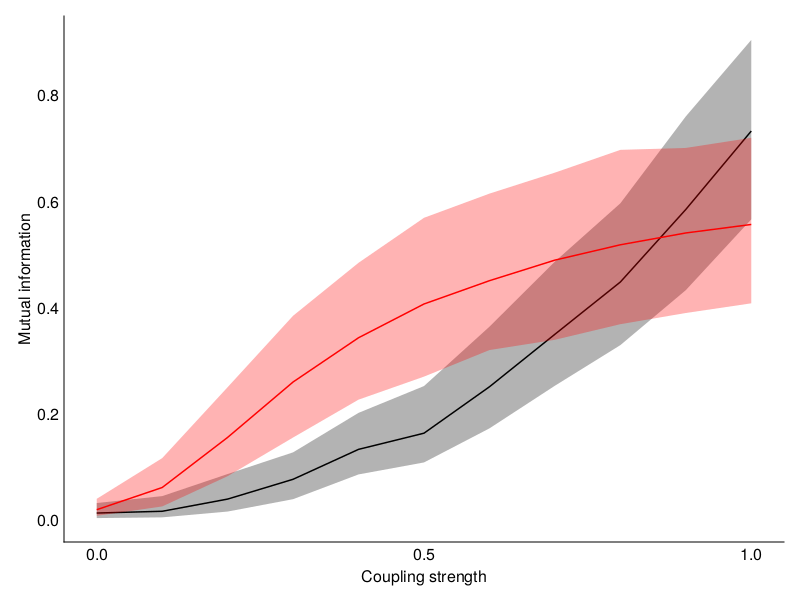As expected, $I(X; Y)$ increases with coupling strength in a system-specific manner.

### Differential MI: Reproducing Kraskov et al. (2004)

Here, we'll reproduce Figure 4 from Kraskov et al. (2004)'s seminal paper on the nearest-neighbor based mutual information estimator. We'll estimate the mutual information between marginals of a bivariate Gaussian for a fixed time series length of 2000, varying the number of neighbors. Note: in the original paper, they show multiple curves corresponding to different time series length. We only show two single curves: one for the KSG1 estimator and one for the KSG2 estimator.

using CausalityTools
using LinearAlgebra: det
using Distributions: MvNormal
using StateSpaceSets: Dataset
using CairoMakie
using Statistics

N = 2000
c = 0.9
Σ = [1 c; c 1]
N2 = MvNormal([0, 0], Σ)
mitrue = -0.5*log(det(Σ)) # in nats
ks = [2; 5; 7; 10:10:70] .* 2

nreps = 30
mis_ksg1 = zeros(nreps, length(ks))
mis_ksg2 = zeros(nreps, length(ks))
for i = 1:nreps
D2 = Dataset([rand(N2) for i = 1:N])
X = D2[:, 1] |> Dataset
Y = D2[:, 2] |> Dataset
measure = MIShannon(; base = ℯ)
mis_ksg1[i, :] = map(k -> mutualinfo(measure, KSG1(; k), X, Y), ks)
mis_ksg2[i, :] = map(k -> mutualinfo(measure, KSG2(; k), X, Y), ks)
end
fig = Figure()
ax = Axis(fig[1, 1], xlabel = "k / N", ylabel = "Mutual infomation (nats)")
scatterlines!(ax, ks ./ N, mean(mis_ksg1, dims = 1) |> vec, label = "KSG1")
scatterlines!(ax, ks ./ N, mean(mis_ksg2, dims = 1) |> vec, label = "KSG2")
hlines!(ax, [mitrue], color = :black, linewidth = 3, label = "I (true)")
axislegend()
fig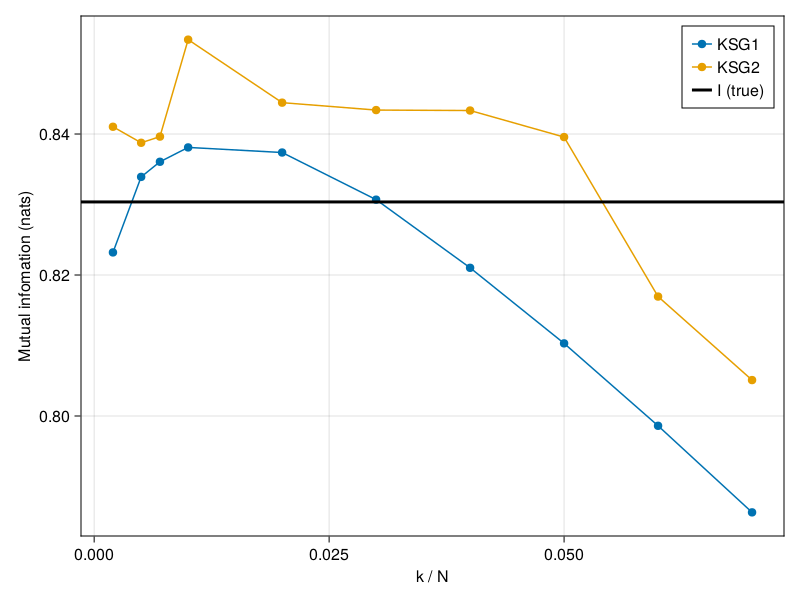### Differential MI: estimator comparison

Let's compare the performance of a subset of the implemented mutual information estimators. We'll use example data from Lord et al., where the analytical mutual information is known.

using CausalityTools
using LinearAlgebra: det
using StateSpaceSets: Dataset
using Distributions: MvNormal
using LaTeXStrings
using CairoMakie

function new_cycle_theme()
# https://nanx.me/ggsci/reference/pal_locuszoom.html
my_colors = ["#D43F3AFF", "#EEA236FF", "#5CB85CFF", "#46B8DAFF",
"#357EBDFF", "#9632B8FF", "#B8B8B8FF"]
cycle = Cycle([:color, :linestyle, :marker], covary=true) # alltogether
my_markers = [:circle, :rect, :utriangle, :dtriangle, :diamond,
:pentagon, :cross, :xcross]
my_linestyle = [nothing, :dash, :dot, :dashdot, :dashdotdot]
return Theme(
fontsize = 22, font="CMU Serif",
colormap = :linear_bmy_10_95_c78_n256,
palette = (
color = my_colors,
marker = my_markers,
linestyle = my_linestyle,
),
Axis = (
backgroundcolor= (:white, 0.2),
xgridstyle = :dash,
ygridstyle = :dash
),
Lines = (
cycle= cycle,
),
ScatterLines = (
cycle = cycle,
),
Scatter = (
cycle = cycle,
),
Legend = (
bgcolor = (:grey, 0.05),
framecolor = (:white, 0.2),
labelsize = 13,
)
)
end

run(est; f::Function, # function that generates data
base::Real = ℯ,
nreps::Int = 10,
αs = [1e-6, 1e-5, 1e-4, 1e-3, 1e-2, 1e-1],
n::Int = 1000) =
map(α -> mutualinfo(MIShannon(; base), est, f(α, n)...), αs)

function compute_results(f::Function; estimators, k = 5, k_lord = 20,
n = 1000, base = ℯ, nreps = 10,
as = 7:-1:0,
αs = [1/10^(a) for a in as])

is = [zeros(length(αs)) for est in estimators]
for (k, est) in enumerate(estimators)
tmp = zeros(length(αs))
for i = 1:nreps
tmp .+= run(est; f = f, αs, base, n)
end
is[k] .= tmp ./ nreps
end

return is
end

function plot_results(f::Function, ftrue::Function;
base, estimators, k_lord, k,
as = 7:-1:0, αs = [1/10^(a) for a in as], kwargs...
)
is = compute_results(f;
base, estimators, k_lord, k, as, αs, kwargs...)
itrue = [ftrue(α; base) for α in αs]

xmin, xmax = minimum(αs), maximum(αs)

ymin = floor(Int, min(minimum(itrue), minimum(Iterators.flatten(is))))
ymax = ceil(Int, max(maximum(itrue), maximum(Iterators.flatten(is))))
f = Figure()
ax = Axis(f[1, 1],
xlabel = "α", ylabel = "I (nats)",
xscale = log10, aspect = 1,
xticks = (αs, [latexstring("10^{$(-a)}") for a in as]), yticks = (ymin:ymax) ) xlims!(ax, (1/10^first(as), 1/10^last(as))) ylims!(ax, (ymin, ymax)) lines!(ax, αs, itrue, label = "I (true)", linewidth = 4, color = :black) for (i, est) in enumerate(estimators) es = string(typeof(est).name.name) lbl = occursin("Lord", es) ? "$es (k = $k_lord)" : "$es (k = $k)" scatter!(ax, αs, is[i], label = lbl) lines!(ax, αs, is[i]) end axislegend() return f end set_theme!(new_cycle_theme()) k_lord = 20 k = 5 base = ℯ estimators = [ Kraskov(; k), KozachenkoLeonenko(), Zhu(; k), ZhuSingh(; k), Gao(; k), Lord(; k = k_lord), KSG1(; k), KSG2(; k), GaoOhViswanath(; k), GaoKannanOhViswanath(; k), ] 10-element Vector{Any}: Kraskov{Int64}(5, 1, 2) KozachenkoLeonenko{Int64}(0, 2) Zhu{Int64}(5, 0, 2) ZhuSingh{Int64}(5, 0, 2) Gao{Int64}(5, 0, 2, true) CausalityTools.Lord(20, 0) KraskovStögbauerGrassberger1{Chebyshev, Chebyshev}(5, 0, Chebyshev(), Chebyshev()) KraskovStögbauerGrassberger2{Chebyshev, Chebyshev}(5, 0, Chebyshev(), Chebyshev()) GaoOhViswanath{Euclidean, Euclidean}(5, 0, Euclidean(0.0), Euclidean(0.0)) GaoKannanOhViswanath(5, 0) #### Family 2 function family2(α, n::Int) Σ = [1 α; α 1] N2 = MvNormal(zeros(2), Σ) D2 = Dataset([rand(N2) for i = 1:n]) X = Dataset(D2[:, 1]) Y = Dataset(D2[:, 2]) return X, Y end function ifamily2(α; base = ℯ) return (-0.5 * log(1 - α^2)) / log(base, ℯ) end αs = 0.05:0.05:0.95 estimators = estimators with_theme(new_cycle_theme()) do f = Figure(); ax = Axis(f[1, 1], xlabel = "α", ylabel = "I (nats)") is_true = map(α -> ifamily2(α), αs) is_est = map(est -> run(est; f = family2, αs, nreps = 20), estimators) lines!(ax, αs, is_true, label = "I (true)", color = :black, linewidth = 3) for (i, est) in enumerate(estimators) estname = typeof(est).name.name |> String scatterlines!(ax, αs, is_est[i], label = estname) end axislegend(position = :lt) return f end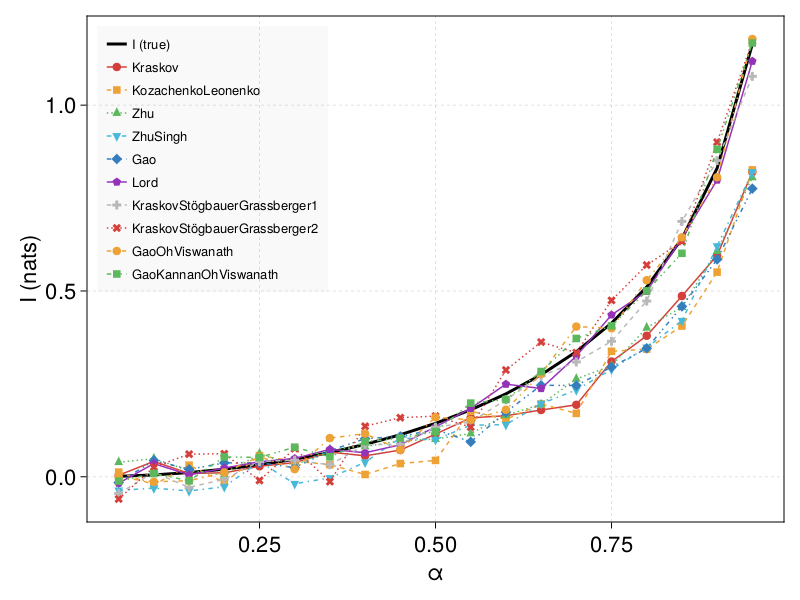#### Family 1 In this system, samples are concentrated around the diagonal$X = Y$, and the strip of samples gets thinner as$\alpha \to 0$. function family1(α, n::Int) x = rand(n) v = rand(n) y = x + α * v return Dataset(x), Dataset(y) end # True mutual information values for these data function ifamily1(α; base = ℯ) mi = -log(α) - α - log(2) return mi / log(base, ℯ) end fig = plot_results(family1, ifamily1; k_lord = k_lord, k = k, nreps = 10, estimators = estimators, base = base)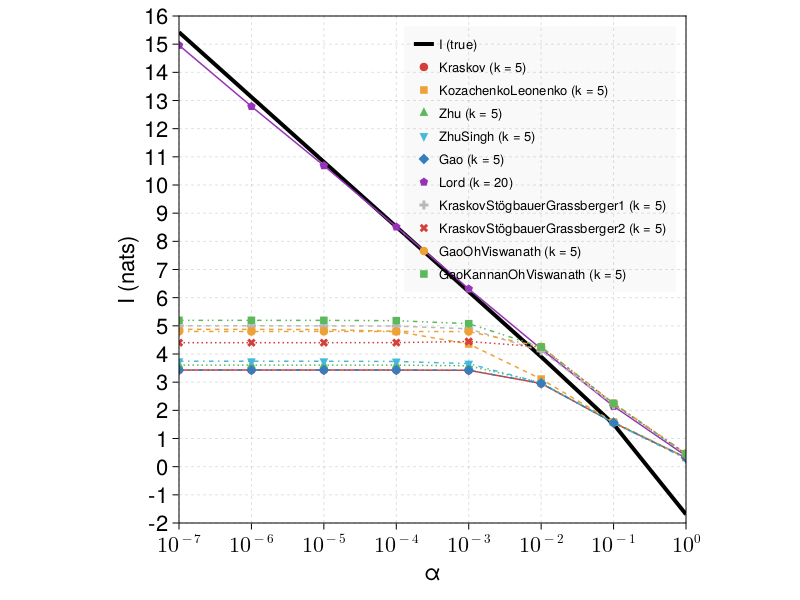#### Family 3 In this system, we draw samples from a 4D Gaussian distribution distributed as specified in the ifamily3 function below. We let$X$be the two first variables, and$Y\$ be the two last variables.

function ifamily3(α; base = ℯ)
Σ = [7 -5 -1 -3; -5 5 -1 3; -1 -1 3 -1; -3 3 -1 2+α]
Σx = Σ[1:2, 1:2]; Σy = Σ[3:4, 3:4]
mi = 0.5*log(det(Σx) * det(Σy) / det(Σ))
return mi / log(base, ℯ)
end

function family3(α, n::Int)
Σ = [7 -5 -1 -3; -5 5 -1 3; -1 -1 3 -1; -3 3 -1 2+α]
N4 = MvNormal(zeros(4), Σ)
D4 = Dataset([rand(N4) for i = 1:n])
X = D4[:, 1:2]
Y = D4[:, 3:4]
return X, Y
end

fig = plot_results(family3, ifamily3;
k_lord = k_lord, k = k, nreps = 10,
n = 2000,
estimators = estimators, base = base)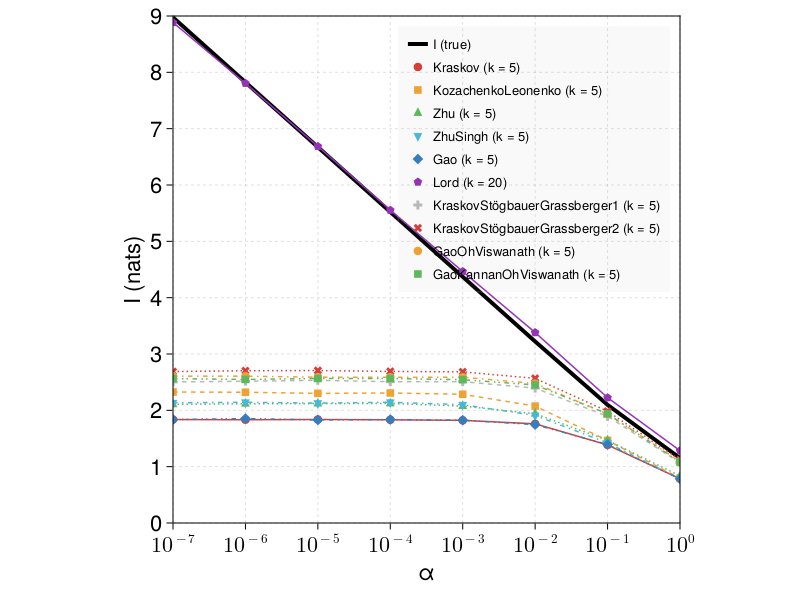We see that the Lord estimator, which estimates local volume elements using a singular-value decomposition (SVD) of local neighborhoods, outperforms the other estimators by a large margin.

### Continuous-discrete MI: estimator comparison

Most estimators suffer from significant bias when applied to discrete data. One possible resolution is to add a small amount of noise to discrete variables, so that the data becomes continuous in practice.

Instead of adding noise to your data, you can consider using an estimator that is specifically designed to deal with continuous-discrete mixture data. One example is the GaoKannanOhViswanath estimator.

Here, we compare its performance to KSG1 on uniformly distributed discrete multivariate data. The true mutual information is zero.

using CausalityTools
using Statistics
using StateSpaceSets: Dataset
using Statistics: mean
using CairoMakie

function compare_ksg_gkov(;
k = 5,
base = 2,
nreps = 15,
Ls = [500:100:1000; 1500; 2000; 3000; 4000; 5000; 1000])

est_gkov = GaoKannanOhViswanath(; k)
est_ksg1 = KSG1(; k)

mis_ksg1_mix = zeros(nreps, length(Ls))
mis_ksg1_discrete = zeros(nreps, length(Ls))
mis_ksg1_cont = zeros(nreps, length(Ls))
mis_gkov_mix = zeros(nreps, length(Ls))
mis_gkov_discrete = zeros(nreps, length(Ls))
mis_gkov_cont = zeros(nreps, length(Ls))

for (j, L) in enumerate(Ls)
for i = 1:nreps
X = Dataset(float.(rand(1:8, L, 2)))
Y = Dataset(float.(rand(1:8, L, 2)))
Z = Dataset(rand(L, 2))
W = Dataset(rand(L, 2))
measure = MIShannon(; base = ℯ)
mis_ksg1_discrete[i, j] = mutualinfo(measure, est_ksg1, X, Y)
mis_gkov_discrete[i, j] = mutualinfo(measure, est_gkov, X, Y)
mis_ksg1_mix[i, j] = mutualinfo(measure, est_ksg1, X, Z)
mis_gkov_mix[i, j] = mutualinfo(measure, est_gkov, X, Z)
mis_ksg1_cont[i, j] = mutualinfo(measure, est_ksg1, Z, W)
mis_gkov_cont[i, j] = mutualinfo(measure, est_gkov, Z, W)
end
end
return mis_ksg1_mix, mis_ksg1_discrete, mis_ksg1_cont,
mis_gkov_mix, mis_gkov_discrete, mis_gkov_cont
end

fig = Figure()
ax = Axis(fig[1, 1],
xlabel = "Sample size",
ylabel = "Mutual information (bits)")
Ls = [100; 200; 500; 1000; 2500; 5000; 10000]
nreps = 5
k = 3
mis_ksg1_mix, mis_ksg1_discrete, mis_ksg1_cont,
mis_gkov_mix, mis_gkov_discrete, mis_gkov_cont =
compare_ksg_gkov(; nreps, k, Ls)

scatterlines!(ax, Ls, mean(mis_ksg1_mix, dims = 1) |> vec,
label = "KSG1 (mixed)", color = :black,
marker = :utriangle)
scatterlines!(ax, Ls, mean(mis_ksg1_discrete, dims = 1) |> vec,
label = "KSG1 (discrete)", color = :black,
linestyle = :dash, marker = '▲')
scatterlines!(ax, Ls, mean(mis_ksg1_cont, dims = 1) |> vec,
label = "KSG1 (continuous)", color = :black,
linestyle = :dot, marker = '●')
scatterlines!(ax, Ls, mean(mis_gkov_mix, dims = 1) |> vec,
label = "GaoKannanOhViswanath (mixed)", color = :red,
marker = :utriangle)
scatterlines!(ax, Ls, mean(mis_gkov_discrete, dims = 1) |> vec,
label = "GaoKannanOhViswanath (discrete)", color = :red,
linestyle = :dash, marker = '▲')
scatterlines!(ax, Ls, mean(mis_gkov_cont, dims = 1) |> vec,
label = "GaoKannanOhViswanath (continuous)", color = :red,
linestyle = :dot, marker = '●')
axislegend(position = :rb)
fig`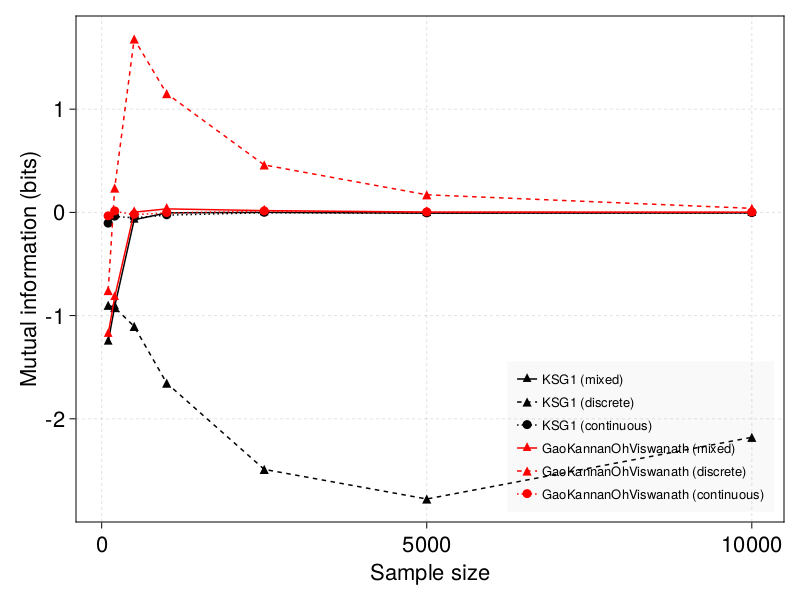• Furuichi2006Furuichi, S. (2006). Information theoretical properties of Tsallis entropies. Journal of Mathematical Physics, 47(2), 023302.
• Martin2004Martin, S., Morison, G., Nailon, W., & Durrani, T. (2004). Fast and accurate image registration using Tsallis entropy and simultaneous perturbation stochastic approximation. Electronics Letters, 40(10), 1.
• Sarbu2014Sarbu, S. (2014, May). Rényi information transfer: Partial Rényi transfer entropy and partial Rényi mutual information. In 2014 IEEE International Conference on Acoustics, Speech and Signal Processing (ICASSP) (pp. 5666-5670). IEEE.
• Jizba2012Jizba, P., Kleinert, H., & Shefaat, M. (2012). Rényi's information transfer between financial time series. Physica A: Statistical Mechanics and its Applications, 391(10), 2971-2989.
• Kraskov2004Kraskov, A., Stögbauer, H., & Grassberger, P. (2004). Estimating mutual information. Physical review E, 69(6), 066138.
• Kraskov2004Kraskov, A., Stögbauer, H., & Grassberger, P. (2004). Estimating mutual information. Physical review E, 69(6), 066138.
• GaoKannanOhViswanath2017Gao, W., Kannan, S., Oh, S., & Viswanath, P. (2017). Estimating mutual information for discrete-continuous mixtures. Advances in neural information processing systems, 30.
• Gao2018Gao, W., Oh, S., & Viswanath, P. (2018). Demystifying fixed k-nearest neighbor information estimators. IEEE Transactions on Information Theory, 64(8), 5629-5661.